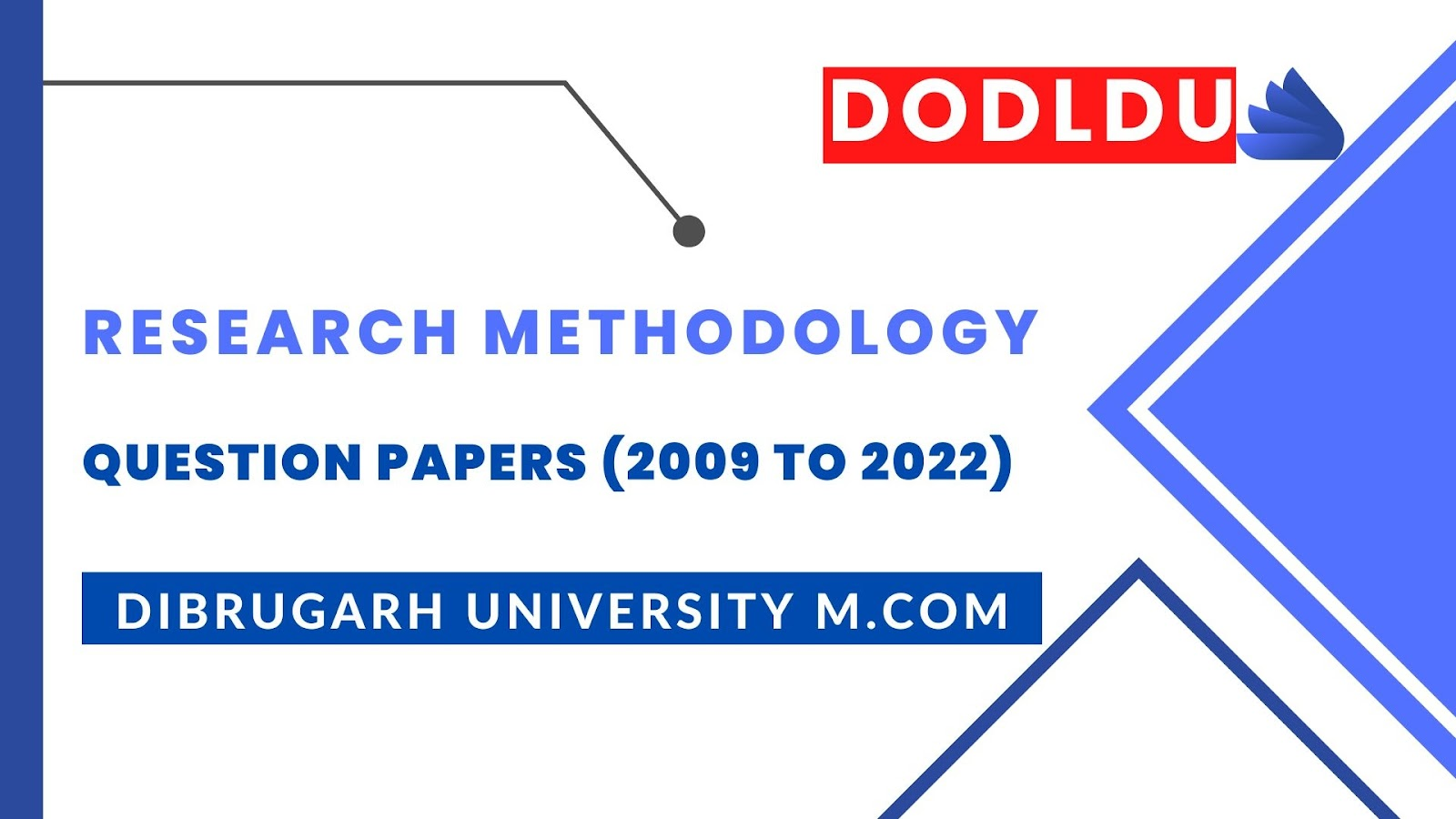# Research Methodology Question Paper 2017 [Dibrugarh University DODLDU M.Com Final Year]

## Research Methodology Question Paper 2017 (Nov-Dec)[Dibrugarh University DODLDU M.Com Final Year]

COMMERCE
Paper: 203
(Research Methodology and Statistical Analysis)
Full Marks: 80
Time: 3 hours
The figures in the margin indicate full marks for the questions
1. (a) The difference in earnings of different income categories is responsible for different buying and usage habits of washing powder. You have been asked to conduct a survey and choose a sample. What kind of sampling will you avail? Reason out your answer. 16
Or
(b) Discuss the difference between ratio scale and interval scale.     16

2. (a) What is data and what are the types of data? Give examples and explain the types of data.        8+8=16
Or
(b) Justify the use of time series analysis in research.     16

3. (a) Discuss on conditional probability and Baye’s Theorem.                      16
Or
(b) Discuss the Statistical Decision Theory.                                                        16

4. (a) What do you understand by testing of hypotheses?                            16
Or
(b) How is sampling distribution done in large and small samples?          16

5. (a) Explain the Wilcoxon signed test.               16
Or
(b) Explain the Kruskal-Walle’s Test.              16
***